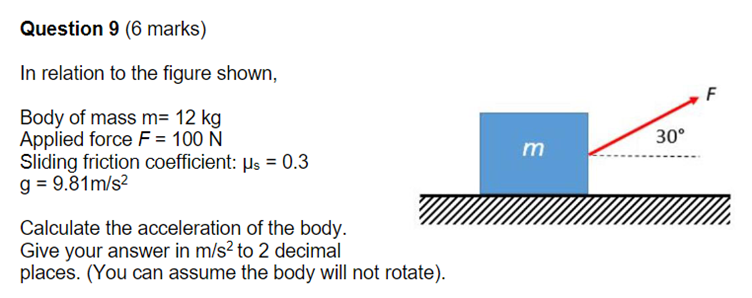# Question Answer is 5.52m/s2. How do i get this answer please ? Question 9 (6 marks) In relation to the figure shown, Body of mass m= 12 kg Applied force F = 100 N Sliding friction coefficient: µs = 0.3 g = 9.81m/s² Calculate the acceleration of the body. Give your answer in m/s² to 2 decimal places. (You can assume the body will not rotate). m 30°WRIWXD The Asker · Mechanical Engineering